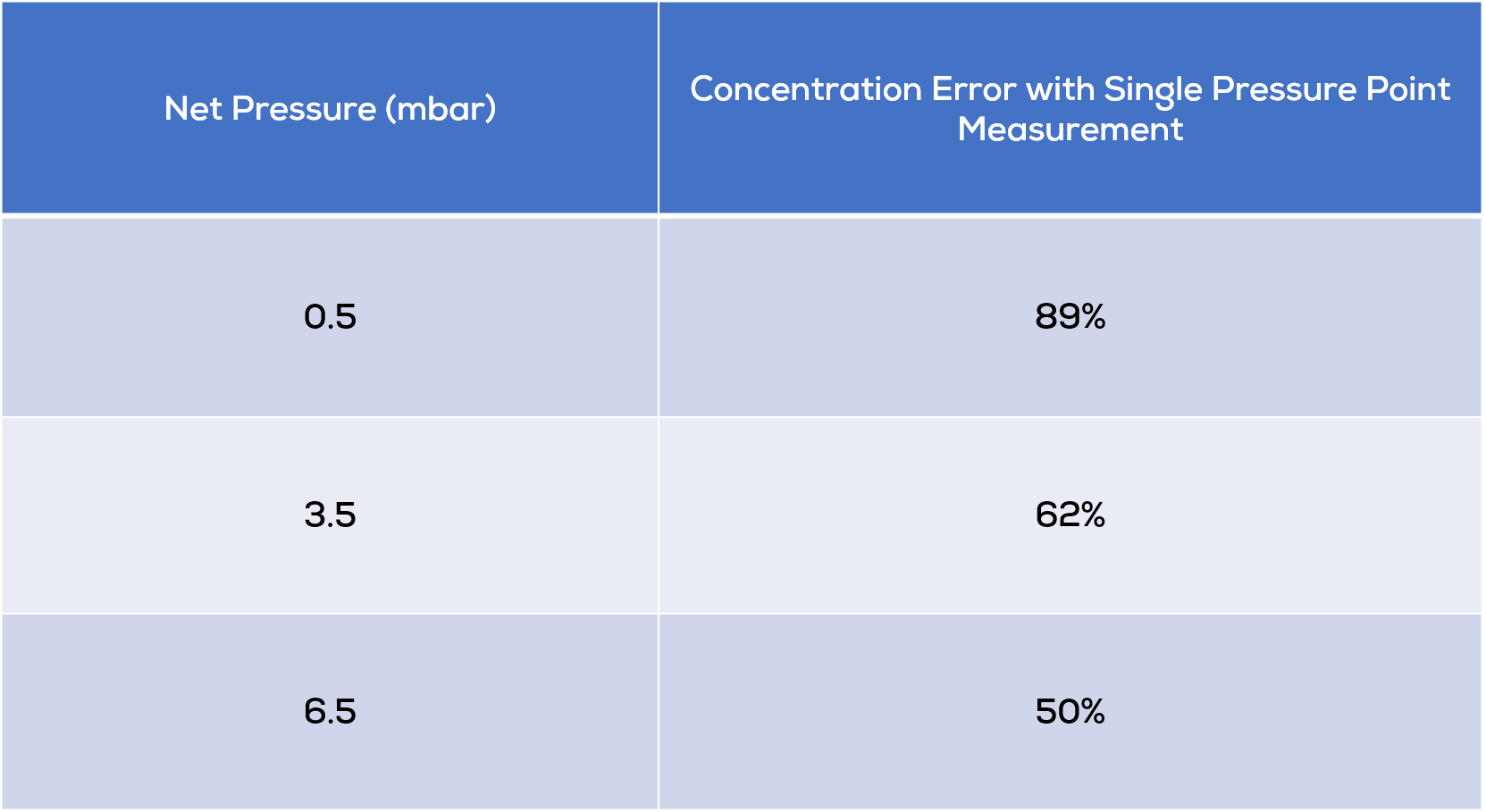1. Izon Science Support Centre
2. TRPS Measurement
3. Sample Preparation and Measurement

# Why do I have to perform multi-pressure measurements?

Multi-pressure measurements give very accurate concentration results by comparing the rate versus pressure gradient of the calibration particles with the rate versus pressure gradient of the sample particles. This eliminates the electrokinetic particle rate and allows particles with different surface charges to be compared.

The calculation of particle concentration at a single differential pressure across the nanopore (i.e. a single fluid flow rate through the nanopore) assumes that the flow of fluid is the only (or vastly dominant) force moving particles through the nanopore.

For larger nanopores with a mid to high pressure differential, this is generally true. For smaller nanopores this is often not true. Electrokinetic forces start to become significant, and the surface charge of the sample particles often differs from that of the calibration particles. By measuring the frequency of blockade peaks at two or more applied pressures (resulting in different flow rates of fluid through the nanopore), Izon’s Control Suite Software is able to calculate the proportion of the blockade peaks that are due to the flow of fluid through the nanopore and hence calculate very accurate relative concentrations of the unknown sample particles.

Figure 1 below shows an example of real data recorded on a small (NP100) nanopore. Two particle sets of similar concentration were measured at three pressures. In this example, calculating the concentration of the 70 nm particles using a single pressure measurement results in a very large error.Figure 1: Multi-pressure concentration analysis.

Single pressure concentration measurements assume a count rate of zero particles at zero pressure. For the plain, uncarboxylated polystyrene particles (100 nm) this is almost always the case, but the highly charged carboxylated particles (70 nm) are passing rapidly through the nanopore due to electrophoresis.

In this example, calculating concentration by comparing the count rates of particles at just one measurement pressure results in a very large error (that decreases with increasing pressure). Table 1 lists typical calculation errors at each of the three pressure sample points shown.

Table 1: Single Pressure Concentration Errors for an NP100 nanopore